### The Penalized Partial Likelihood Approach for Fitting Frailty Models

Let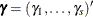be the vector of random components for the s clusters.

#### Gamma Frailty Model

Assume each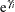has an independent and identically distributed gamma distribution with mean 1 and a common unknown variance; that is,is iid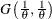. The penalty function is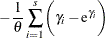plus a function of. The penalized partial log likelihood is given by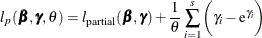where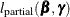is the log of any of the partial likelihoods in the sections Partial Likelihood Function for the Cox Model and The Multiplicative Hazards Model.

The profile marginal log-likelihood of this shared frailty model (Therneau and Grambsch, 2000, pp. 257–258) is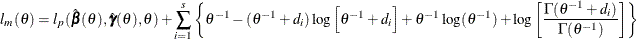where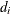is the number of events in the ith cluster.

The maximization of this approximate likelihood is a doubly iterative process that alternates between the following two steps:

• For a provisional value of, the best linear unbiased predictors (BLUP) ofand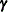are computed by maximizing the penalized partial log-likelihood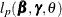. The marginal likelihood is evaluated. This constitutes the inner loop.

• A new value ofis obtained by the golden section search based on the marginal likelihood of all the previous iterations. This constitutes the outer loop.

The outer loop is iterated until the bracketing interval ofis small.

#### Lognormal Frailty Model

With each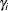having a zero-mean normal distribution and a common variance, the penalty function is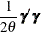plus a function of. The penalized partial log likelihood is given by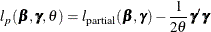where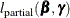is the log of any of the partial likelihoods in the sections Partial Likelihood Function for the Cox Model and The Multiplicative Hazards Model.

For a given, letbe the negative Hessian of the penalized partial log likelihood; that is,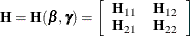where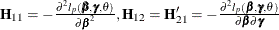, and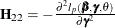.

The marginal log likelihood of this shared frailty model is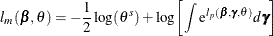Using a Laplace approximation to the integral as in Breslow and Clayton (1993), an approximate marginal log likelihood (Ripatti and Palmgren, 2000; Therneau and Grambsch, 2000) is given by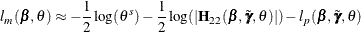The maximization of this approximate likelihood is a doubly iterative process that alternates between the following two steps:

• For a provisional value of, PROC PHREG computes the best linear unbiased predictors (BLUP) ofandby maximizing the penalized partial log likelihood. This constitutes the inner loop.

• Forandfixed at the BLUP values, PROC PHREG estimatesby maximizing the approximate marginal likelihood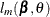. This constitutes the outer loop.

The outer loop is iterated until the difference between two successive estimates ofis small.

The ML estimate ofis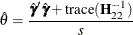The variance for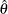is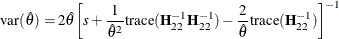The REML estimation ofis obtained by replacing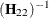by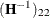.

The inverse of the finalmatrix is used as the variance estimate of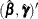.

The final BLUP estimates of the random components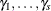can be displayed by using the SOLUTION option in the RANDOM statement. Also displayed are estimates of the lognormal frailties, which are the exponentiated estimates of the BLUP estimates.

#### Wald-Type Tests for Penalized Models

Letbe the negative Hessian of the partial log likelihood: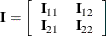where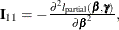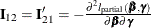, and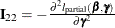. Write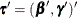. The Wald-type chi-square statistic for testing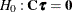is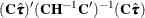Letbe the negative Hessian of the penalized partial log likelihoodat the ML estimate; that is,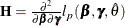. Let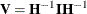. Gray (1992) recommends the following generalized degrees of freedom for the Wald test: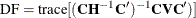See Therneau and Grambsch (2000, Section 5.8) for a discussion of this Wald-type test.

PROC PHREG uses the label "Adjusted DF" to represent this generalized degrees of freedom in the output.